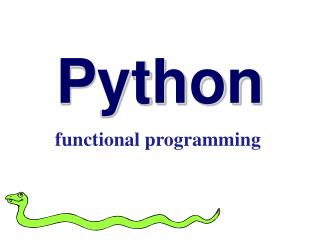DownloadDownload PresentationPython

# Python

Download Presentation## Python

- - - - - - - - - - - - - - - - - - - - - - - - - - - E N D - - - - - - - - - - - - - - - - - - - - - - - - - - -
##### Presentation Transcript

1. Python functional programming

2. Functions are first-class objects Functions can be used as any other datatype, eg: • Arguments to function • Return values of functions • Assigned to variables • Parts of tuples, lists, etc >>>defsquare(x): return x*x >>>defapplier(q, x): return q(x) >>> applier(square, 7) 49

3. Lambda Notation Python’s lambda creates anonymous functions >>> lambda x: x + 1 <function <lambda> at 0x1004e6ed8> >>> f = lambda x: x + 1 >>> f <function <lambda> at 0x1004e6f50> >>> f(100) 101

4. Lambda Notation Be careful with the syntax >>> f = lambda x,y: 2 * x + y >>> f <function <lambda> at 0x87d30> >>> f(3, 4) 10 >>> v = lambda x: x*x(100) >>> v <function <lambda> at 0x87df0> >>> v = (lambda x: x*x)(100) >>> v 10000

5. Lambda Notation Limitations • Note: only one expression in the lambda body; Its value is always returned • The lambda expression must fit on one line! • Lambda will probably be deprecated in future versions of python Guido is not a lambda fanboy

6. Functional programming Python supports functional programming idioms Builtins for map, reduce, filter, closures, continuations, etc. These are often used with lambda

7. Example: composition >>> def square(x): return x*x >>> def twice(f): return lambda x: f(f(x)) >>> twice <function twice at 0x87db0> >>> quad = twice(square) >>> quad <function <lambda> at 0x87d30> >>> quad(5) 625

8. Example: closure >>> def counter(start=0, step=1): x = [start] def _inc(): x += step return x return _inc >>> c1 = counter() >>> c2 = counter(100, -10) >>> c1() 1 >>> c2() 90

9. map >>> def add1(x): return x+1 >>> map(add1, [1,2,3,4]) [2, 3, 4, 5] >>> map(lambda x: x+1, [1,2,3,4]) [2, 3, 4, 5] >>> map(+, [1,2,3,4], [100,200,300,400]) map(+,[1,2,3,4],[100,200,300,400]) ^ SyntaxError: invalid syntax

10. map • + is an operator, not a function • We can define a corresponding add function >>> def add(x, y): return x+y >>> map(add,[1,2,3,4],[100,200,300,400]) [101, 202, 303, 404] • Or import the operator module >>> from operator import * >>> map(add, [1,2,3,4], [100,200,300,400]) [101, 202, 303, 404] >>> map(sub, [1,2,3,4], [100,200,300,400]) [-99, -198, -297, -396]

11. filter, reduce • Python has buiting for reduce and filter >>> reduce(add, [1,2,3,4]) 10 >>> filter(odd, [1,2,3,4]) [1, 3] • The map, filter and reduce functions are also at risk 